RFK2B
 Name:    RFK2B

Multiple Choice
Identify the choice that best completes the statement or answers the question.

1.

Put the syllables together and choose the right picture.  Foot-ball
 a.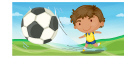c.b.2.

How many syllables are there in the word watermelon?
 a. 2 c. 1 b. 3 d. 4

3.

How many syllables are there in the word sad?
 a. 2 c. 1 b. 3 d. 4

4.

How many syllables are there in the word basket?
 a. 2 c. 1 b. 3 d. 4

5.

How many syllables are there in the word window?
 a. 3 c. 5 b. 2 d. 4

6.

Put the syllables together and choose the right picture.  Ap-ple
 a.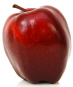c.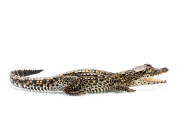b.7.

Put the syllables together and choose the right picture.  Di-no-saur
 a.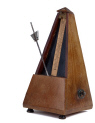c.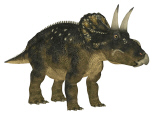b.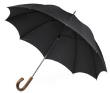8.

Put the syllables together and choose the right picture.  Pen-cil
 a.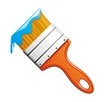c.b.9.

Put the syllables together and choose the right picture.  Cup-cake
 a.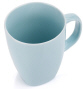c.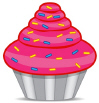b.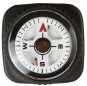10.

How many syllables are there in the word pinecone.
 a. 1 c. 3 b. 2 d. 4